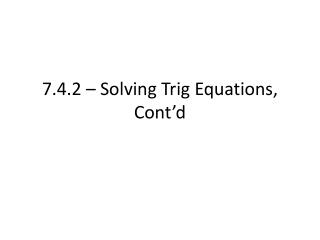DownloadDownload Presentation7.4.2 – Solving Trig Equations, Cont’d

# 7.4.2 – Solving Trig Equations, Cont’d

Download Presentation## 7.4.2 – Solving Trig Equations, Cont’d

- - - - - - - - - - - - - - - - - - - - - - - - - - - E N D - - - - - - - - - - - - - - - - - - - - - - - - - - -
##### Presentation Transcript

1. 7.4.2 – Solving Trig Equations, Cont’d

2. Sometimes, we may have more than one trig function at play while trying to solve • Like having two variables

3. >1 trig function • When we have more than one trig function, we want to try and simplify the equation in terms of a single trig function • How? • Use identities • Expand or factor using algebra • Write in terms of sine and cosine, combine • Look for any like terms to cancel

4. Example. Solve the equation sin2x + cos2x + tan2x = 0.

5. Example. Solve the equation cosx – 1 = sinx • Hint: treat the left side as a binomial.

6. Verifying Solutions • Similar to algebra, we must be able to verify that solutions of particular equations are accurate • Options: • 1) Plug in, pull values from table • 2) Use calculator, be careful of typing answers in

7. Example. Verify for the equation that x = 2π/3 is a solution to 2 cosx + 1 = 0.

8. Using your calculator • Sometimes, finding exact solutions may not be feasible • In this event, we will jump to using our calculators, and treating them as an algebraic expression

9. To use your calculator: • 1) Write the function with all terms on a single side • 2) Plug in into your “graph” section • 3) Select an appropriate range • 4) Use the “find-zero” feature we have used before

10. Example. Estimate the solutions to the equation x tan(x) – 3 = 0 on the interval [0, 2π)

11. Example. Estimate the solutions to the equation 2 sin x = 1 – 2 cos x on the interval [0, 2π)

12. Assignment • Pg. 586 • 13, 17, 33, 39, 42, 45